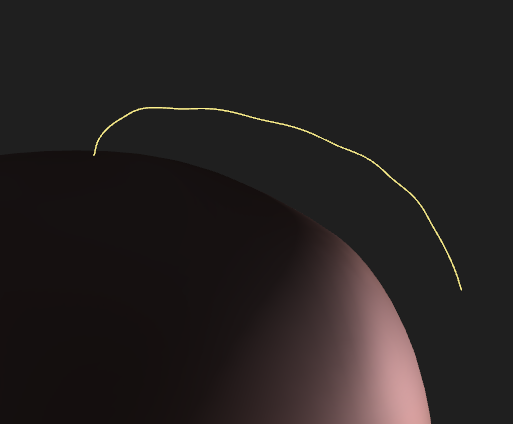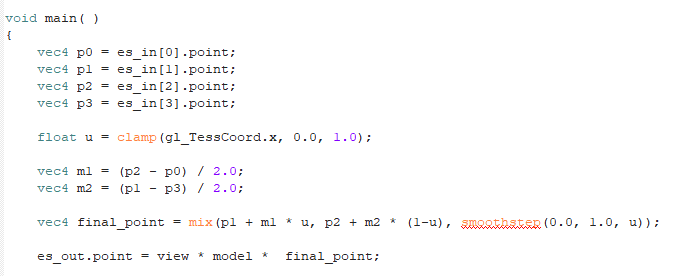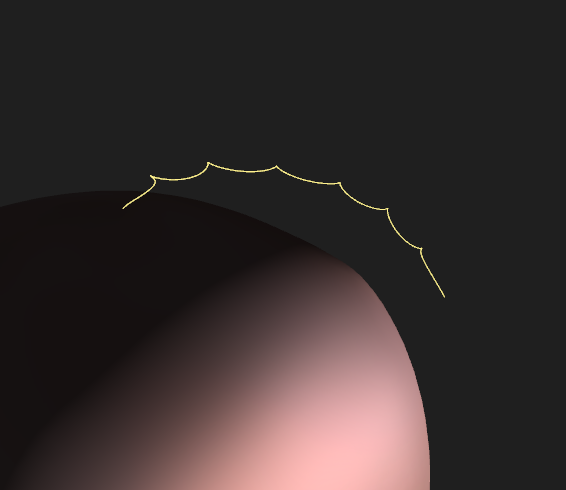# B-Spline Curve Using Tessellation Shader

I would like to create smooth curves using the tessellation shader. First, I tried to create Bezier curves with tessellation (patch number = 4), but if I have a line with more than 4 control points, then there are gaps at every 4 vertices. This is why it is recommended to use B-splines. This is the B-spline formula, where Pi is the control point i:

(u is just `gl_TessCoord.x`)

So if we have 8 control points for example, then we would need:
P0 P1 P2 P3
P1 P2 P3 P4
P2 P3 P4 P5 etc.

However, the tessellation stage takes 4 vertices at a time, which means it would take:
P0 P1 P2 P3
P4 P5 P6 P7

There is no overlap in the way the tessellator takes the vertices, correct? If that’s true then how am I going to create the B-splines?

One solution would be instead of using the initial buffer:
[P0 , P1 , P2 , P3 , P4 , P5 , P6 ,P7]

to pass this buffer (new control points):
[P0 , P1 , P2 , P3 , P1 , P2 , P3 , P4 , P2 , P3 , P4 , P5 … ]

But isn’t that a waste of space, because the new buffer has more than 2x the size of the initial control points?

Um… that’s not why people use B-splines. If you don’t have continuity between your Bezier control points, that’s because you’ve done the math wrong. You’re probably taking every set of 4 control points as distinct, rather than each sequential 4 control points. That is, 8 control points do not yield two curves; they yield 5.

That is, the problem you’re encountering generating B-splines is the same as the Bezier problem.

That’s entirely up to you and how you choose to feed in the vertex data.

Define “waste”. The actual position data has not changed. You simply have more indices. But you have more indices because you are trying to render more patches.

It isn’t a “waste” if it’s a thing you have to do.

Also, indices can be 16-bit integers, while positions are often multiple 4-byte floats.

The math were correct, but as you said I took 4 distinct points every time instead of repeating the vertices so that’s what was causing the gaps.

So the only option is to repeat vertices when I send them to the vertex shader. I thought maybe there could be another way but it seems like this is the only option. That’s what I called “waste” initially because I thought there could be a way to prevent vertex repetition but it doesn’t look like there is.

I couldn’t find anywhere implementations of B-splines using the tessellation shader with more than 2-4 points. I found only a few about Hermite curves and Catmull-Rom splines but as I said they only focused on a very small number of points.

You only need to repeat the indices. The vertex shader output is cached so if the processed vertex is in the cache the vertex shader won’t be re-run.

1 Like

Thank you both. I still have some questions thoughI implemented this in the tessellation shader and let’s say that I have 8 control points. Then the index buffer should be:
0123 1234 2345 3456 4567 according to this site

However, after vertex 3 (0123), we draw back to vertex 1 (1234) and these two shouldn’t be connected.

An implementation I’m actually following describes the algorithm as:

I’m a bit confused as to what Pi Pi+1 etc. will be for a practical example.

If you evaluate that expression at u=0 and u=1, you’ll see that

P(0) = (1/6)P[i] + (4/6)P[i+1] + (1/6)P[i+2]

P(1) = (1/6)P[i+1] + (4/6)P[i+2] + (1/6)P[i+3]

So P(1) for one segment is equal to P(0) for the next segment.

P(0) is most strongly influenced by P[i+1] while P(1) is most strongly influenced by P[i+2].

Also, note that the derivatives at the endpoints are P’(0)=(P-P)/2 and P’(1)=(P-P)/2 (so if a sequence of vertices lie in a straight line, the curve will follow the line).

In general, B-spline curves don’t interpolate (pass through) the vertices, but approximate them.

At the ends of the curve, you don’t have a “previous” or “next” vertex, so you use the first or last vertex twice. For 8 control points (7 segments), the indices would be:

0012 0123 1234 2345 3456 4567 5677

If you were to a draw line between the second and third vertex of each segment (01 12 23 … 67), you’d get a polyline which approximates the curve.

I searched a bit more and I found this implementation and everything you said about derivatives and interpolating to draw a line, makes much more sense now. Here is the test result for a test line with 8 control points:The line seems a bit shaky but I guess it’s because of how the original control points were drawn?

Here is the TES for anyone who stumbles upon this thread:My mistake was that I thought only the matrix calculations were enough. It didn’t even cross my mind to use derivatives and interpolation. I will need to take a good look at the B-spline math again. Thank you!

Or because the calculations are completely different. For a start, the resulting polynomial is quartic rather than cubic, so the second derivative will change quadratically rather than linearly across the segment.

Ok so I did the math this time and here are the results for 0, 1st and 2nd derivate when u=0 and u=1:

0 derivative

``````vec4 m1 = (1.0 / 6.0) *p0 + (4.0 / 6.0) * p1 + (1.0 / 6.0) * p2;
vec4 m2 = (1.0 / 6.0) *p1 + (4.0 / 6.0) * p2 + (1.0 / 6.0) * p3;
``````1st derivative

``````vec4 m1 = (p2 - p0) / 2.0;
vec4 m2 = (p1 - p3) / 2.0;
``````2nd derivative

``````vec4 m1 = p0 - 2.0*p1 + p2;
vec4 m2 = p1 - 2.0*p2 + p3;
``````Am I missing something here?The issue is essentially that higher-degree polynomials tend to oscillate more. Cubics are preferred because that’s the lowest degree which can satisfy all four constraints (position and first derivative at start and end points).

Have you tried comparing to the actual B-spline formula from your first post?

1 Like

Yep! Problem solved now.So I think this is the definitive TES code (not the other one I posted with the 1st derivative):

``````float b0 = (-1.0*u*u*u + 3.0*u*u - 3.0*u + 1.0) * (1.0/6.0);
float b1 = (3.0*u*u*u - 6.0*u*u + 4.0 ) * (1.0/6.0);
float b2 = (-3.0*u*u*u + 3.0*u*u + 3.0*u + 1.0) * (1.0/6.0);
float b3 = (u*u*u) * (1.0/6.0);

es_out.point =  view * model * (b0*p0 + b1*p1 + b2*p2 + b3*p3);
``````

I could even create a `mat4` and pass it as a uniform instead of writing every basis function by hand.

Again, thank you!